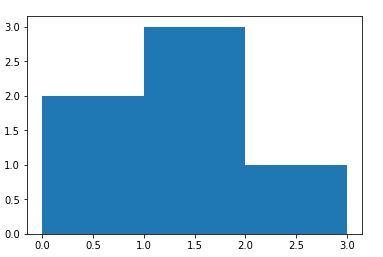﻿ NumPy: Compute the histogram of nums against the bins - w3resource# NumPy: Compute the histogram of nums against the bins

## NumPy Statistics: Exercise-14 with Solution

Write a NumPy program to compute the histogram of nums against the bins.

Sample Solution:-

Python Code:

``````import numpy as np
import matplotlib.pyplot as plt
nums = np.array([0.5, 0.7, 1.0, 1.2, 1.3, 2.1])
bins = np.array([0, 1, 2, 3])
print("nums: ",nums)
print("bins: ",bins)
print("Result:", np.histogram(nums, bins))
plt.hist(nums, bins=bins)
plt.show()
```
```

Sample Output:

```nums:  [0.5 0.7 1.  1.2 1.3 2.1]
bins:  [0 1 2 3]
Result: (array([2, 3, 1], dtype=int64), array([0, 1, 2, 3]))```

Python Code Editor:

Have another way to solve this solution? Contribute your code (and comments) through Disqus.

What is the difficulty level of this exercise?

Test your Python skills with w3resource's quiz

﻿

## Python: Tips of the Day

Chain Operators

You can chain comparison operators in Python as following: n = 10 result = 1 < n < 20

```distance = 200
checker = 100 < distance < 350

print(checker)
```

Output:

`True`